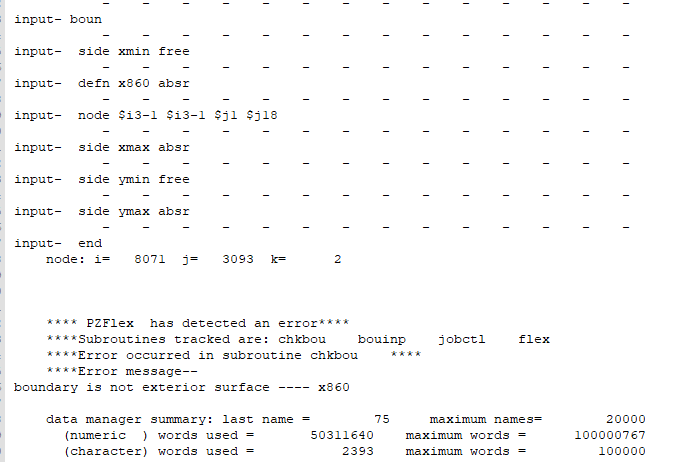# The BC is not exterior surface 回答済み

Hi，

The picture is my BC . The print file says boundary is not exterior surface and k =2.But my model is 2D.The k should be equal  to 0.Why does it say k=2 when the BC is set like this?If I set the BC to following,the absorbing boundary doesn't absorb ultrasonic and will report  'bad value' error.

`boun	side xmin free		side xmax absr	side ymin free	side ymax absrend	`

How should I set the BC?

Best regards，

Enclosed here is the node list.

`          x1                   (  real   ) =    0.000000              x2                   (  real   ) =   0.8000000E-03          x3                   (  real   ) =   0.8608000              x4                   (  real   ) =   0.8618000              idx                  ( integer ) =          4          xmax                 (  real   ) =   0.8618000              y1                   (  real   ) =    0.000000              y2                   (  real   ) =   0.1625000E-01          y3                   (  real   ) =   0.3625000E-01          y4                   (  real   ) =   0.4625000E-01          y5                   (  real   ) =   0.6625000E-01          y6                   (  real   ) =   0.7625000E-01          y7                   (  real   ) =   0.9625000E-01          y8                   (  real   ) =   0.1062500              y9                   (  real   ) =   0.1262500              y10                  (  real   ) =   0.1362500              y11                  (  real   ) =   0.1562500              y12                  (  real   ) =   0.1662500              y13                  (  real   ) =   0.1862500              y14                  (  real   ) =   0.1962500              y15                  (  real   ) =   0.2162500              y16                  (  real   ) =   0.2262500              y17                  (  real   ) =   0.2462500              jaend                ( integer ) =         17          y18                  (  real   ) =   0.3300000              jdx                  ( integer ) =         18          ymax                 (  real   ) =   0.3300000              i1                   ( integer ) =          1          i2                   ( integer ) =          9          i3                   ( integer ) =       8072          i4                   ( integer ) =       8081          indgrd               ( integer ) =       8081          j1                   ( integer ) =          1          j2                   ( integer ) =        153          j3                   ( integer ) =        341          j4                   ( integer ) =        435          j5                   ( integer ) =        622          j6                   ( integer ) =        716          j7                   ( integer ) =        903          j8                   ( integer ) =        997          j9                   ( integer ) =       1184          j10                  ( integer ) =       1278          j11                  ( integer ) =       1465          j12                  ( integer ) =       1559          j13                  ( integer ) =       1746          j14                  ( integer ) =       1840          j15                  ( integer ) =       2027          j16                  ( integer ) =       2121          j17                  ( integer ) =       2308          j18                  ( integer ) =       3093          jndgrd               ( integer ) =       3093`

### 3件のコメント

• Absorbin boundary conditions need to be placed on the extents/exterior/limits of the model you have specified the nodal location i3-1 looking at your node map i4 lies on the exterior of the model.

• I understand.But the node i3 is the boudary of the geometry and the i4 is the boundary of the model.If I  set both of them absr, it the i3 absorbing boundary doesn't absorb ultrasonic and will report  'bad value' error.

• What model is this for we are currently looking at or plan to look at other models you have shared, just to gie me a better understanding of the variables you posted above

サインインしてコメントを残してください。